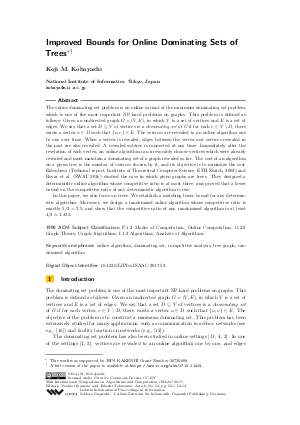Document# Improved Bounds for Online Dominating Sets of Trees

### Author Koji M. Kobayashi## File

LIPIcs.ISAAC.2017.52.pdf
• Filesize: 0.5 MB
• 12 pages

## Cite As

Koji M. Kobayashi. Improved Bounds for Online Dominating Sets of Trees. In 28th International Symposium on Algorithms and Computation (ISAAC 2017). Leibniz International Proceedings in Informatics (LIPIcs), Volume 92, pp. 52:1-52:12, Schloss Dagstuhl - Leibniz-Zentrum für Informatik (2017)
https://doi.org/10.4230/LIPIcs.ISAAC.2017.52

## Abstract

The online dominating set problem is an online variant of the minimum dominating set problem, which is one of the most important NP-hard problems on graphs. This problem is defined as follows: Given an undirected graph G = (V, E), in which V is a set of vertices and E is a set of edges. We say that a set D \subseteq V of vertices is a dominating set of G if for each v \in V \setminus D, there exists a vertex u \in D such that {u, v} \in E. The vertices are revealed to an online algorithm one by one over time. When a vertex is revealed, edges between the vertex and vertices revealed in the past are also revealed. A revelaed subtree is connected at any time. Immediately after the revelation of each vertex, an online algorithm can choose vertices which were already revealed irrevocably and must maintain a dominating set of a graph revealed so far. The cost of an algorithm on a given tree is the number of vertices chosen by it, and its objective is to minimize the cost. Eidenbenz (Technical report, Institute of Theoretical Computer Science, ETH Zurich, 2002) and Boyar et al. (SWAT 2016) studied the case in which given graphs are trees. They designed a deterministic online algorithm whose competitive ratio is at most three, and proved that a lower bound on the competitive ratio of any deterministic algorithm is two. In this paper, we also focus on trees. We establish a matching lower bound for any deterministic algorithm. Moreover, we design a randomized online algorithm whose competitive ratio is at most 5/2 = 2.5, and show that the competitive ratio of any randomized algorithm is at least 4/3 \approx 1.333.
##### Keywords
• online algorithm
• dominating set
• competitive analysis
• tree graph
• randomized algorithm

## Metrics

• Access Statistics
• Total Accesses (updated on a weekly basis)
0

## References

1. A. Borodin and R. El-Yaniv. Online Computation and Competitive Analysis. Cambridge University Press, 1998.2. J. Boyar, S. J. Eidenbenz, L. M. Favrholdt, M. Kotrbčik, and K. S. Larsen. Online dominating set. In Proc. of the 15th Scandinavian Symposium and Workshops on Algorithm Theory, pages 21:1-21:15, 2016.3. D.-Z. Du and P.-J. Wan. Connected Dominating Set: Theory and Applications. Springer, 2013.4. S. J. Eidenbenz. Online dominating set and variations on restricted graph classes. Technical report, Institute of Theoretical Computer Science, ETH Zürich, 2002.5. F. V. Fomin, D. Kratsch, and G. J. Woeginger. Exact (exponential) algorithms for the dominating set problem. In Proc. of the 30th international conference on Graph-Theoretic Concepts in Computer Science, pages 245-256, 2004.6. F.V. Fomin, F. Grandoni, and D. Kratsch. Some new techniques in design and analysis of exact (exponential) algorithms. In Bulletin of the EATCS, pages 47-77, 2005.7. W. Goddard and M. A. Henning. Independent domination in graphs: A survey and recent results. Discrete Mathematics, 313(7):839-854, 2013.8. F. Grandoni. Independent domination in graphs: A survey and recent results. Journal of Discrete Algorithms, 4(2):209-214, 2006.9. M. Henning and A. Yao. Total Domination in Graphs. Springer, 2013.10. Y. Iwata. A faster algorithm for dominating set analyzed by the potential method. In Proc. of the 6th international conference on Parameterized and Exact Computation, pages 41-54, 2011.11. G. H. King and W. G. Tzeng. On-line algorithms for the dominating set problem. Information Processing Letters, 61:11-14, 1997.12. F. Plastria. Static competitive facility location: An overview of optimisation approaches. European Journal of Operational Research, 129(3):461-470, 2001.13. I. Schiermeyer. Exact algorithms for dominating set. Discrete Applied Mathematics, 156(17):3291-3297, 2008.14. D. D. Sleator and R. E. Tarjan. Amortized efficiency of list update and paging rules. Communications of the ACM, 28(2):202-208, 1985.15. J. M. M. van Rooij and H. L. Bodlaender. Exact algorithms for dominating set. Discrete Applied Mathematics, 159(17):2147-2164, 2011.16. J. Y. Yu and P. H. J. Chong. A survey of clustering schemes for mobile ad hoc networks. IEEE Communications Surveys and Tutorials, 7(1):32-48, 2005.X

Feedback for Dagstuhl Publishing